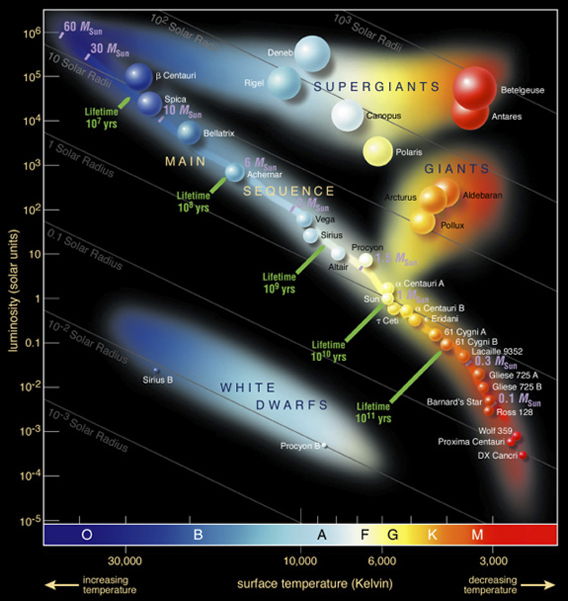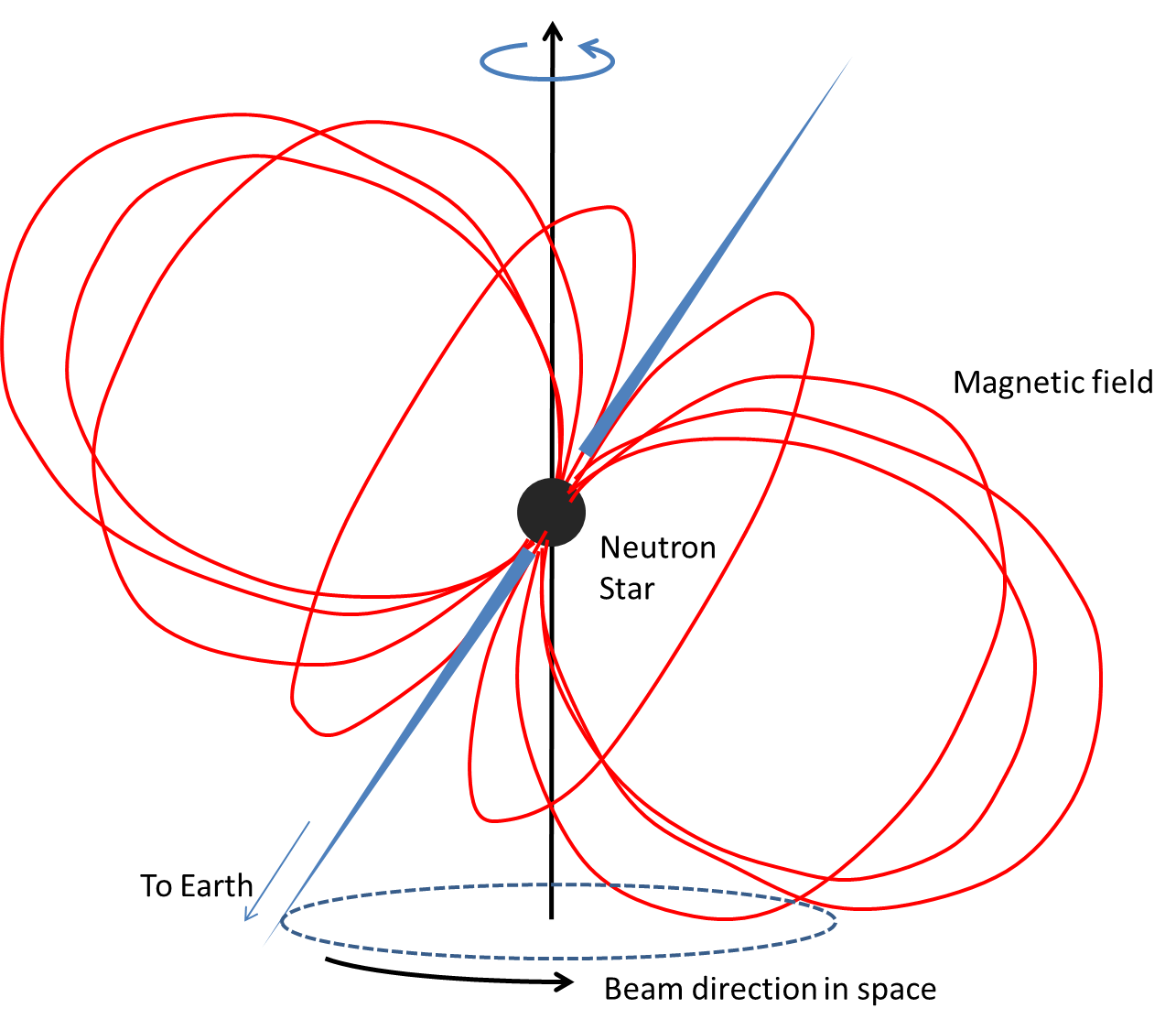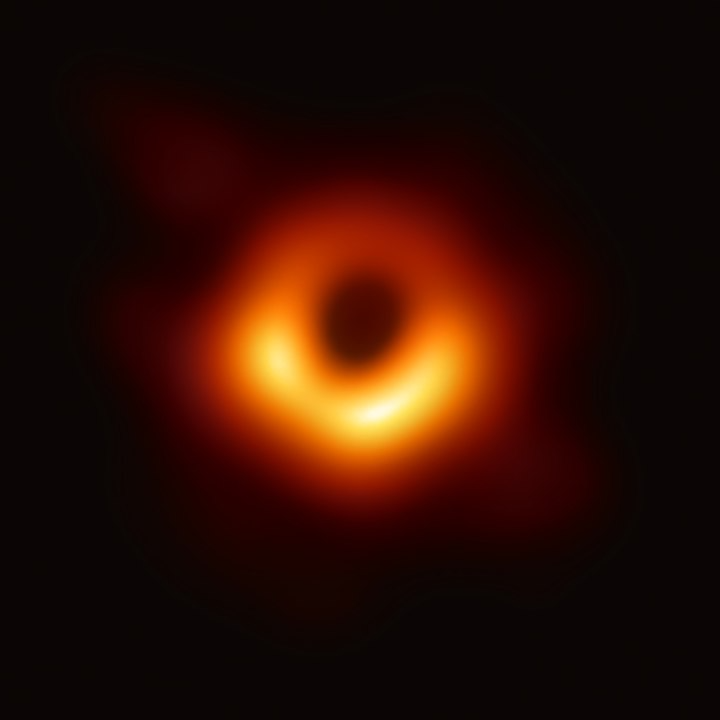## Section59.5Life Cycles of Stars

A star is born when gravity pulls together clouds of material in the interstellar medium. The force of gravity tries to collapse the star while the pressure of the gas and radiation inside the star counteracts the force of gravity. The two forces remain in a hydrostatic equilibrium for much of the life of a star. The heat and radiation in the interior of a star is provide by various nuclear reactions.

Initially, stars get their energy from fusing hydrogen into helium, as is presently done by the Sun. The stars where this is the dominant source of energy are in the main-sequence line in the Hertzsprung-Russel (H-R) Diagram reprodeuced here in Figure 59.5.1.Figure 59.5.1. The Hertzsprung-Russel Diagram identifying many well known stars in the Milky Way galaxy. Credits: This photograph was produced by European Southern Observatory (ESO) and released under Creative Commons.

When there is not much hydrogen left in the center, the star contracts which increases the pressure sufficiently to cause the fusion of helium into carbon at the center and that of hydrogen into helium in a shell around the center. The star then moves up and right in the H-R diagram as it evolves away from the main sequence. Subsequent nuclear burning produces an iron-rich core. Recall that the energy per nucleon is highest near iron and adding more nucleons to iron requires more energy rather than release of energy.

Eventually, the star runs out of energy sources and it begins to collapse. The initial collapse in the core is preveneted from collapsing the star completely due to the degeneracy pressure caused by the quantum requirement of Pauli's exclusion principle. Pauli's exclusion principle states that no two electrons (or fermions) can occupy the same quantum state.

Collapsing the star brings fermions into the same physical area where no more than two electrons, one with up spin and the other with down spin can exist. This causes an upward pressure called the degenracy pressure which supports the gravitational force up to a limit called, the Chandrasekhar limit If the mass of the collapsing material is more than about 1.4 solar mass, the Chandrasekhar limit, the degeneracy pressure cannot prevent collapse. In that case, the star continues to collapse. If the mass of the star is less than the Chandrasekhar's limit, the star just dies after collapsing into a small ball of electrons. This type of star is called white dwarf

For more massive stars than 1.4 solar masses, the pressure from gravity is more than the degeneracy pressure and the star continues to collapse. With increase in pressure a;nd temperature in the core it becomes possible to cause reaction between protons and electrons to make neutrons and antineutrinos

\begin{equation*} \textrm{p} + \textrm{e}^{-} \rightarrow\ \textrm{n} + \nu_e. \end{equation*}

The core of the star turns into neutrons. The collapsing material releases enormous amount of gravitational energy which causes a spectacular explosion, called supernova The explosion of supernova releases neutrinos and light. The light from one such supernova in a galaxy masks all other stars in the host galaxy. The pressure at the center in a supernova explosion is so great that all the elements above iron are formed during these explosions and with the explosion they are dispersed in the interstellar space. All the other elements found on Earth and else where were made in supernovae.

### Subsection59.5.1Neutron Stars

At the end of the supernova explosion, the core left is a very dense star which consists mostly of neutrons. The remnant is called a neutron star whose gravitational collapse is supported by the degeneracy pressure of neutrons, just as white dwarf is supported by the degeneracy pressure of electrons. The mass of a neutron star is comparable to that of the Sun, but its radius is only about $10\text{ km}$ or so. Due to the conservation of angular momentum and due to the fact of explosion being radially out, the angular momentum of the original star ends up as the angular momentum of the neutron star. Since the moment of inertia of the neutron star is considerably smaller than the original star, it rotates at enormous angular speed.

\begin{equation*} I_{0} \omega _{0} = I_{NS} \omega_{NS},\ \ I_{NS} \ll I_{0} \\ \Longrightarrow\ \ \omega_{NS} >> \omega _{0} . \end{equation*}

Neutrons have magnetic dipole moment also. As a result, a neutron star has an enormous magnetic field, about a trillion times stronger than Earth's magnetic. In 1967 Franco Pacini suggested that if the neutron stars were spinning and had large magnetic fields, then electromagnetic waves would be emitted. A search of intense electromagnetic source in radio waves led to the discovery of pulsars by Jocelyn Bell, a student of Anthony Hewish, in England in 1967.

Pulsars are neutron stars whose axis of rotation is misaligned with respect to the magnetic poles such that the electromagnetic waves emitted from the magnetic poles come in the view of the Earth and appear as if some one is turning light on and off with a definite period, akin to the light from the light house. The pulsar detected by Bell and Hewish had a period of 1.337302088331 second and pulse width of 0.04 second. A schematic view of the rotation of neutron star leading to observation of pulses on Earth is illustrated in Figure 59.5.2.Figure 59.5.2. Schematic view of a pulsar/neutron star. The emission from the neutron star is at an angle to the rotation axis so that the beam from the star comes towards the once per period.

### Subsection59.5.2Black Holes

If the supernova was from the evolutionary end point of a star with mass more than 10 times the mass of the Sun, the degenracy pressure from neutrons may not be enough to support the gravitational collapse of the remnant star. The star continues to collapse to a very dense compact object of nearly zero volume. The gravity around such a compact object is so strong that even light cannot escape its pull. Such an object is called a black hole.

The center of the Milky Way galaxy is supposed to be a black hole. The pull of the black hole is especially strong within a radius of the black hole, called event horizon. The distance from the center of the black hole to the event horizon is equal to the \textbf{Schwarzscild radius}\index{Schwarzscild radius} of the black hole. For a black hole of mass M the Schwarzschild radius is give by

\begin{equation} r_s = \frac{2G_N M}{c^2}.\label{eq-schwarzschild-radius}\tag{59.5.1} \end{equation}

Einstein's theory of gravity shows that no information from within the event horizon, $r \lt r_S\text{,}$ of a black hole can reach outside world. For an object of the mass of the mass of the Sun the Schwarzschild radius is about $3\text{ km}\text{.}$ Although the $r_s$ of the Sun is much less than the radius of the Sun, the $r_s$ of a black hole is larger than the radius of the blackhole due to the collapse to almost zero size.

If a black hole is formed near another star, then the material from the companion star will be sucked into the balck hole. The falling material will be ionized and heated to millions of degrees and therefore will emit X rays. The source of X ray binaries have yielded several candidates for black holes. One such candidate is the V404 Cygni binary star system which has a black hole of mass about 12 solar masses with the companion star slightly less mass than the mass of the Sun from which the black hole is accreting matter.

In 2019, The Event Horizon Telescope (EHT) researchers releaed an image of a supermassive black hole and its shadow in the centre of Messier 87. This is displyed in Figure 59.5.3.Figure 59.5.3. A supermassive black hole and its shadow in the centre of Messier 87. Image by Event Horizon Telescope (EHT).